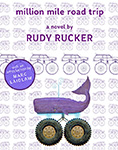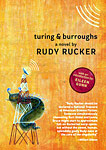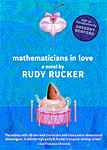Click covers for info. Copyright (C) Rudy Rucker 2021.

## What Shape Is the Scrooge Cubic Acre?

I finished writing my novel Juicy Ghosts this week. Now I’m going to the beach or lying around at home reading Uncle Scrooge comics. And I’ve invented a math puzzle, described in this post, and solved by Bob Hearn on February 21, 2021. Some may term this post fanatical and unreadable but, hey, there’s some nice illos anyway! And don’t worry too much about the details of the math. Just let it soak in through your skin.The Scrooge Cubic Acre

Uncle Scrooge’s money bin is approximately a cube that’s 100 feet on each edge, making a volume of a 1,000,000 cubic feet, as a million is a hundred cubed.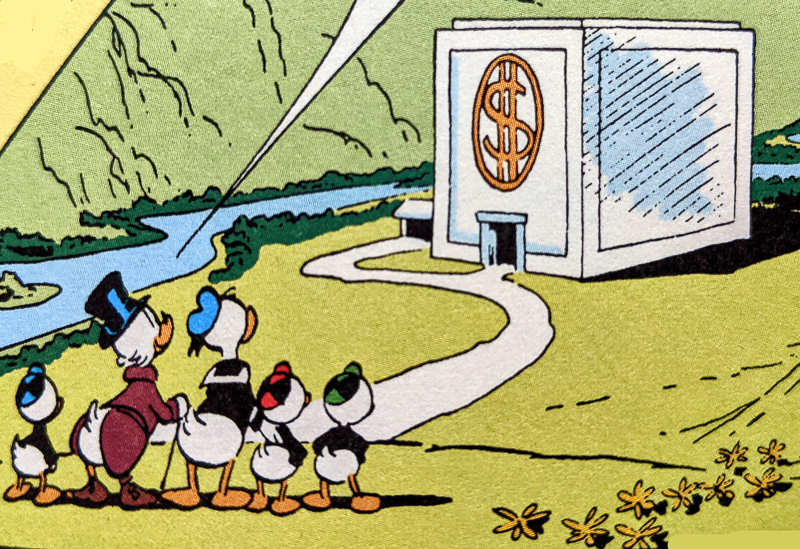Scrooge frequently says it holds three cubic acres. Therefore a cubic acre must be a third of a million cubic feet, that is about 333,333 cubic feet. Call this quantity the Scrooge cubic acre.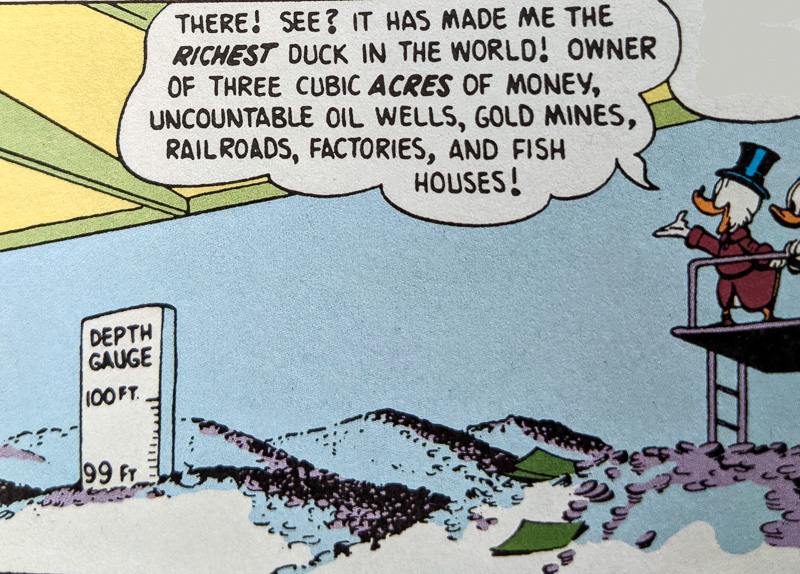The mystery is this. Nobody except Uncle Scrooge has ever talked about “cubic acres.” The unit has no defined meaning. An acre is in fact a measure of area and not volume. Specifically, it’s 43,560 square feet.

I propose that we define the Scrooge Cubic Acre to be a 3D geometrical solid with these two properties:

I. Its volume is a third of a million square feet.
II. Its surface area is an acre.

What might the a Scrooge Cubic Acre look like? Let’s explore some options.A Spherical Cubic Acre?

Let’s look at a sphere whose surface area is an acre.

In terms of radius R, the surface area S of a sphere is 4 pi R^2.
Conversely, R is the square root of S / 4 pi.
[Note that pi is our old friend 3.141, and we can round 4 pi to 12.6.]

So if S is an acre, that is, 43,560 sq. ft., then r is about 59 feet.
The volume V of a sphere with radius Rr is 4/3 pi R^3.
So a sphere whose surface area is an acre will have a volume of 851,247 cu. ft.

But this much too big to match the desired Scrooge Cubic Acre volume of 333,333 cu. ft.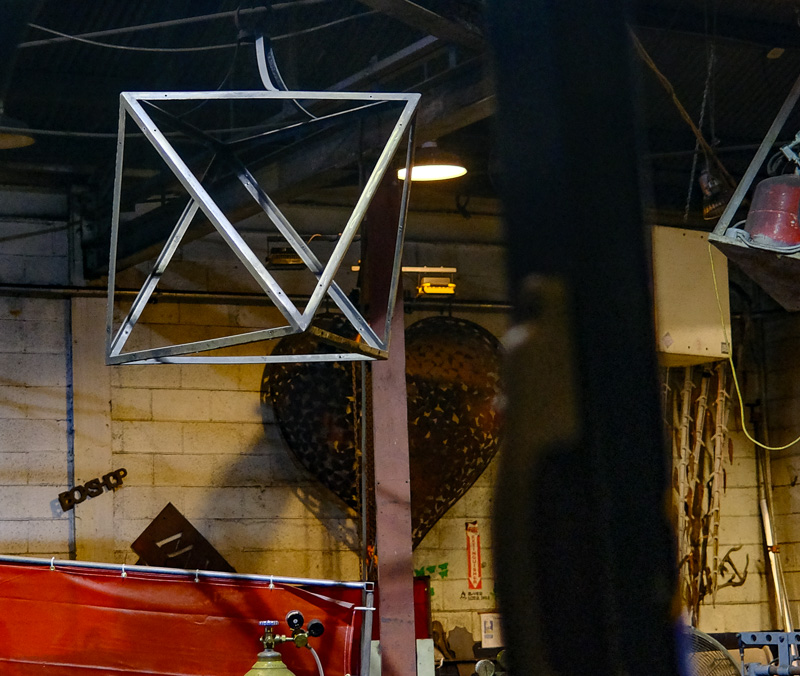A Cubical Cubic Acre?

Let’s whittle down the volume-holding ability of our “cubic acre” shape from sphere to cube. Let’s check out the volume of a cube whose surface area is an acre.

In terms of edge length E, the surface area S of a cube is 6 x E^2. Conversely E is the square root of (S / 6).

So if S is an acre, then E is about 85 feet, and the volume e is 85^3, or 620,724 cubic feet. This is almost twice as big as the targeted Scrooge Cubic Acre volume of 333,333 cubic feet.

What would happen if we didn’t include the base of the cube surface in its surface area? E would be the square root of (S/5), which would be even bigger, so the volume would be even bigger, so that’s no good.Pyramid Cubic Acre

We’ll drop down to a still less voluminous shape for our Scrooge Cubic Acre. We’ll use—of course!—a square-based pyramid, a classic shape, sometimes seen in Scrooge’s adventures.

Note that there is no standard height for a square-based pyramid. So we can in fact diddle with this, and end up with the volume and surface area we want.

We have a pyramid of a surface area S and volume V.
For the pyramid’s dimensions we can specify the square base’s edge as E. And, when convenient, we can write SE for the semiedge E/2.
The altitude A of the pyramid is the height of the peak above the base.
The slant height H of the pyramid is the shortest length up along one face of the pyramid to the summit.Background facts:
SE^2 + A^2 = H^2 by the Pythagorean theorem.
A pyramid’s volume of 1/3 is its base area times its altitude.
The surface area is the base square plus the four isosceles face-triangles.
A triangle’s area is 1/2 times base times height.

For a Scrooge Cubic Acre pyramid we have a Volume equation and a Surface Equation. Plus a H Replacement equation that let’s us replace H by a formula in A and E.

(Volume)  V = 1/3 x E^2 x A.  And we require V to be 333,000 cu. ft.
(Surface)  S = E^2 + 2 x E x H. And we require X to be 43,650 sq. ft.
(H Replacement) H^2 = 0.25 E^2 + A^2
(A Replacement) A^2 = H^2 – 0.25 E^2

Do we include the base square of the pyramid in the surface area or not? Better to include it, as our goal is to get a smaller volume for a given area.

So fine, we have a range of “pyramid cubic acre” shapes. There are many pyramids with the desired volume of a third of a million cubic feet. We only need to find the one with a one-acre surface area. We can shift among them, by changing the the ratio between A and E.

For simplicity, let’s start with the kind of pyramid you get if you dissect a cube into six square pyramids who touch at their tips at the hidden center of the cube. In this dissected cube pyramid,” A is E/2 or what we also call SE.For this proposed cubic acre pyramid:
V = 1/3 x E^2 x E/2 = 1/6 E^3.
E = cube root of 6 V
H = sqrt (SE^2 + SE^2) = sqrt(2)*SE

If the size of V is to be that of a Scrooge Cubic Acre, that is, 333,333 cu ft, then E would be the cube root of 6 x V, which crunches out to 125 ft, which seems like a nice, reasonable size. And the A would be half of that, or 62.5 feet. And the slant height H would be sqrt(2) times that, or 88 ft.

Is the surface area of this dissected pyramid cubic acre in any way close to that of an actual acre of 43,560 sqrt ft? Well, let’s check. It’s 37,656 sq. ft.

Getting there!Now you need to tweak it a little. You want to get a bit more surface area out of that same volume. This means making the pyramid be flatter, more spread out, but without changing its volume. You we can do this by pushing down on its apex, thus reducing the altitude and increasing the edge length and thinking of the volume inside an an incompressible fluid.(1) What are the precise E and A values that work?

(2) Does their ratio bear any relationship to the Golden Proportion?

(Solution to 1) To solve for the valued of E and A, use the H Replacement equation to remove H from the Surface equation. Then use the Volume equation solve for A in terms of E. Then remove A from the Surface equation by substituting the equivalent formula in E. And you end up with a quartic equation in E. I got stuck on that, but then Bob Hearn stepped in.

The quartic has only E^4 and E^2 in it, so, Hearn points out, it can be thought of as quadratic formula in E^2, and you can solve for E^2 with the usual quadratic formula. Upshot? Hearn tells us that E = 139.3383263, A = 51.50606676. Or, rounding off, it’s close to a 139 foot edge and an altitude of 51 feet. A nice reasonable pyramid with a volume of a third of a million cubic feet and a surface are of an acre!

(Cryptic Addendum by Hearn) What is boils down to is E²S² – 2E⁴S = 36V²

(Comment on 2) And no, it bears no relation to the golden proportion…no real reason it should.

(Gratuitous off topi comment) Should a cubic acre be thought of as a six-dimensional polytope? [No. What do you think I am? A science fiction writer?]

(Further step). How about making the Scrooge Cubic acre be a flattish cone instead of a square pyramid. What would be the radius R and the altitude A?